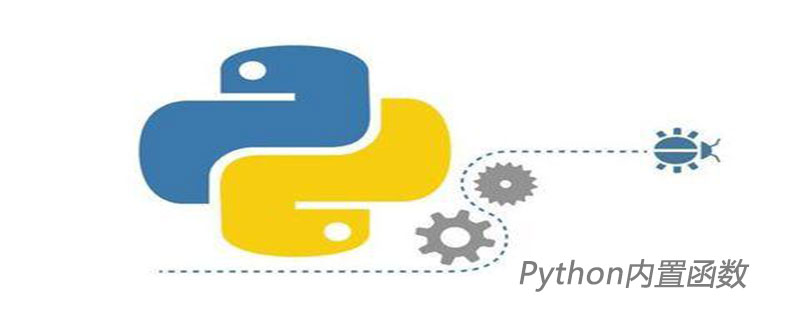Jquery中文网 www.jquerycn.cn
Jquery中文网 >  Python编程  >  Python入门  >  正文 一分钟学会如何查看Python内置函数的用法及其源码

# 一分钟学会如何查看Python内置函数的用法及其源码

jquery中文网为您提供一分钟学会如何查看Python内置函数的用法及其源码等资源，欢迎您收藏本站，我们将为您提供最新的一分钟学会如何查看Python内置函数的用法及其源码资源```import math
dir(math)```

```['__doc__',
'__name__',
'__package__',
'__spec__',
'acos',
'acosh',
'asin',
'asinh',
'atan',
'atan2',
'atanh',
'ceil',
'copysign',
'cos',
'cosh',
'degrees',
'e',
'erf',
'erfc',
'exp',
'expm1',
'fabs',
'factorial',
'floor',
'fmod',
'frexp',
'fsum',
'gamma',
'gcd',
'hypot',
'inf',
'isclose',
'isfinite',
'isinf',
'isnan',
'ldexp',
'lgamma',
'log',
'log10',
'log1p',
'log2',
'modf',
'nan',
'pi',
'pow',
'sin',
'sinh',
'sqrt',
'tan',
'tanh',
'tau',
'trunc']```

```import math
help(math)``````import random
random.__file__```

`'D:\\Anaconda2\\envs\\py3\\lib\\random.py'`

***.pyc的文件是编译后的文件，打开是看不懂的，所以要看***.py文件。

```def expovariate(self, lambd):
"""Exponential distribution.
lambd is 1.0 divided by the desired mean.  It should be
nonzero.  (The parameter would be called "lambda", but that is
a reserved word in Python.)  Returned values range from 0 to
positive infinity if lambd is positive, and from negative
infinity to 0 if lambd is negative.
"""
# lambd: rate lambd = 1/mean
# ('lambda' is a Python reserved word)

# we use 1-random() instead of random() to preclude the
# possibility of taking the log of zero.
return -_log(1.0 - self.random())/lambd```
[]